Practice the questions of McGraw Hill Math Grade 4 Answer Key PDF Chapter 2 Test to secure good marks & knowledge in the exams.

Identify the property shown in each number sentence.
Question 1.
0 + 3, 240 = 3,240
Commutative property.
0 + 3, 240 = 3,240.

Explanation:
Commutative property: When we add two or more whole numbers, their sum is the same regardless of the order of the addends.
Sum of:
0 + 3, 240 = 3,240.

Question 2.
22,055 + 672 = 672 + 22,055
22,055 + 672 = 672 + 22,055 = 22,727.
Commutative property.

Explanation:
Commutative property: When we add two or more whole numbers, their sum is the same regardless of the order of the addends.
Sum of:
22,055 + 672 = 672 + 22,055
= 22,727.

Question 3.
16 + (0 + 33) = 16 + 33
16 + (0 + 33) = 16 + 33 = 49.
Associative property.

Explanation:
Associative property.
When three or more numbers are added, the sum is the same regardless of the grouping of the addends.
Sum of:
16 + (0 + 33)
= 16 + 33
= 49.

Question 4.
(13 + 1) + 7 = 13 + (1 + 7)
(13 + 1) + 7 = 13 + (1 + 7) = 21.
Associative property.

Explanation:
Associative property.
When three or more numbers are added, the sum is the same regardless of the grouping of the addends.
Sum of:
(13 + 1) + 7 = 13 + (1 + 7)
= 13 + 8
= 21.

Question 5.
(4 + 9) + 69 = 4 + (9 + 69)
(4 + 9) + 69 = 4 + (9 + 69) = 82.
Associative property.

Explanation:
Associative property.
When three or more numbers are added, the sum is the same regardless of the grouping of the addends.
Sum of:
(4 + 9) + 69 = 4 + (9 + 69)
= 4 + 78
= 82.

Question 6.
18 + 40 = 40 + 18
18 + 40 = 40 + 18 = 58.
Commutative property.

Explanation:
Commutative property: When we add two or more whole numbers, their sum is the same regardless of the order of the addends.
Sum of:
18 + 40 = 40 + 18 = 58.

Question 7.
Janet tells her father that when zero is added to a number, the number does not change. She states that the rule is called the Commutative Property of Addition. Is she correct? Why or why not?
No, she is not correct because when zero is added to a number, the number does not change, its additive identity property not Commutative Property of Addition.

Explanation:
It means that additive identity is “0” as adding 0 to any number, gives the sum as the number itself.
Commutative property of addition: Changing the order of addends does not change the sum.

Question 8.
Which subtraction problem would you use to check the answer to 145 + 83 = 228? Place a check mark next to your answer.
____ 145 – 83 ____ 228 – 83 ____ 83 + 228 ____ 83 + 145
145 + 83 = 83 + 145 = 228.

Explanation:
145 – 83 = 62.
228 – 83 = 145.
83 + 228 = 311.
83 + 145 = 228. *

Question 9.
Which addition problem would you use to check the answer to 598 — 266 = 332? Place a check mark next to your answer.
____ 598 + 332 ____ 266 + 598 ____ 598 + 266 ____ 332 + 266
598 – 266 = 332.
=> 332 = 598 – 266.*

Explanation:
598 + 332 = 930.
266 + 598 = 864.
598 + 266 = 864.
332 + 266 = 598.
=> 332 = 598 – 266.*

Question 10.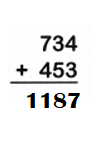Explanation:
Sum of:
734 + 453 = 1,187.

Question 11.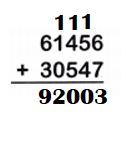Explanation:
Sum of:
61,456 + 30,547 = 92,003.

Question 12.Explanation:
Sum of:
53,406 + 6,732 = 60,138.

Question 13.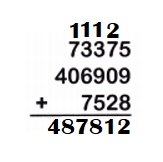Explanation:
Sum of:
73,375 + 4,06,909 + 7,528
= 4,80,284 + 7,528
= 4,87,812.

Question 14.Explanation:
Sum of:
4,41,775 + 4,10,963
= 8,52,738.
Question 15.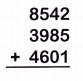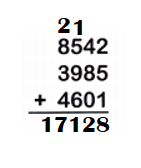Explanation:
Sum of:
8,542 + 3,985 + 4,601
= 12,527 + 4,601
= 17,128.

Subtract
Question 16.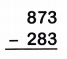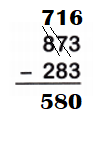Explanation:
Difference between:
873 – 283
= 590.

Question 17.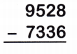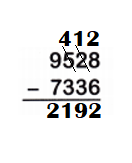Explanation:
Difference between:
9,528 – 7,336
= 2,192.

Subtract.
Question 18.Explanation:
Difference between:
48,016 – 25,632
= 22,384.

Question 19.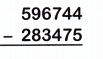Explanation:
Difference between:
5,96,744 – 2,83,475
= 3,13,269.

Question 20.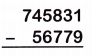Explanation:
Difference between:
7,45,831 – 56,779
= 6,89,052.

Question 21.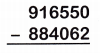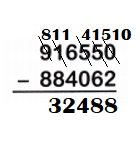Explanation:
Difference between:
9,16,550 – 8,84,062
= 32,488.

Add or subtract to complete each table.
Question 22.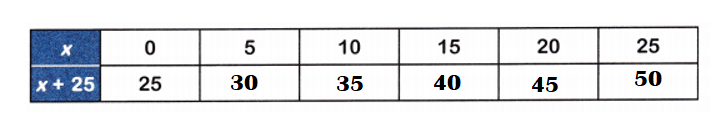Explanation:
x = 5
=> x + 25 = 5 + 25 = 30.
x = 10
=> x + 25 = 10 + 25 = 35.
x = 15
=> x + 25 = 15 + 25 = 40.
x = 20
=> x + 25 = 20 + 25 = 45.
x = 25
=> x + 25 = 25 + 25 = 50.

Question 23.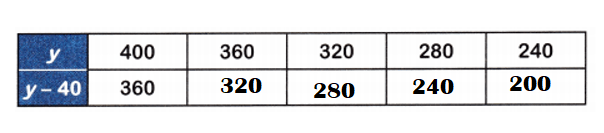Explanation:
y = 400.
=> y – 40 = 400 – 40 = 360.
y = 360.
=> y – 40 = 360 – 40 = 320.
y = 320.
=> y – 40 = 320 – 40 = 280.
y = 280.
=> y – 40 = 280 – 40 = 240.
y = 240.
=> y – 40 = 240 – 40 = 200.

Question 24.
Gavin is 13 years old. His older cousin is 27 years old. When Gavin is 46 years old, how old will his cousin be?
Number of years old his older cousin after = 60.

Explanation:
Number of years old Gavin = 13.
Number of years old his older cousin = 27.
Number of years old Gavin after = 46.
Difference:
Number of years old Gavin after – Number of years old Gavin
= 46 – 13
= 33.
Number of years old his older cousin after = 33 + Number of years old his older cousin
= 33 + 27
= 60.

Question 25.
Megan spent 930 minutes last month swimming, and she spent 1,395 minutes doing homework. If she spends 1,240 minutes this month swimming, how many minutes will she spend doing homework if she increases the time spent by the same amount?
Number of minutes she spends doing homework this month = 2,635.

Explanation:
Number of minutes Megan spent last month swimming = 930.
Number of minutes Megan spent doing homework = 1,395.
Number of minutes Megan spent this month swimming = 1,240.
Number of minutes she spends doing homework this month = Number of minutes Megan spent this month swimming + Number of minutes Megan spent doing homework
= 1,240 + 1,395
= 2,635.

Question 26.
On Saturday, 11,794 people watched a rock concert at a stadium. On Sunday, 2,455 fewer people watched. About how many people watched a concert at the stadium on Sunday?